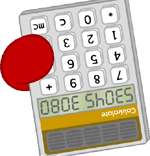# Mr SpeakerHexadecimal sure comes in handy sometimes. Like, say, um, if you wanted to know why there was some of the alphabet on your scientific calculator for example. There's been a few times I've needed the following functions to convert decimal bytes to hex in javascript: converting decimal RGB values to those #FF00FF looking ones, or far more commonly, creating XBM images by hand. You do create your XBM images by hand don't you?

First up, here's a javascript object I use to convert bytes (0-255) between the two numbering systems. I can't remember where I got the guts of the dec2hex function. But I do like it:

``````
var HexConverter = {
hexDigits : '0123456789ABCDEF',

dec2hex : function( dec )
{
return( this.hexDigits[ dec >> 4 ] + this.hexDigits[ dec & 15 ] );
},

hex2dec : function( hex )
{
return( parseInt( hex, 16 ) )
}
}
``````

Here's a couple of example usages:

`alert( HexConverter.dec2hex( 240 ) ); // gives: F0`
`alert( HexConverter.hex2dec( 'EF' ) ); // gives: 239`

That's all you need for byte conversion happiness. But if you, like me, want some hardcore performance boostin' you'll want to use a lookup table. I got the lookup table method from the impressive Wolf5k. The lookup table means that you don't have to do CPU intensive operations every time you need to convert decimal to hex. Yeah, it's not really CPU intensive. But if you want to make Wolfenstein in 5k of javascript you'll need all the help you can get.

``````
var HexHelper = {
hexDigits : '0123456789ABCDEF',

lookup : [],
initLookup : function()
{
for( var i = 0; i < 256; i++ )
{
this.lookup[ i ] = '0x' + this.hexDigits[ i >> 4 ] + this.hexDigits[ i & 15 ];
}
},
}
HexHelper.initLookup();
``````

example usage:

`alert( HexHelper.lookup ); //gives 0x81`

All this talk of XBM images has made me sad. I miss them. DAMN YOU MICROSOFT!